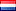﻿ Super Mechs - Free Games - Flash Ghetto

# Super Mechs

• Current rating 3.37/5
• 1
• 2
• 3
• 4
• 5

Rates: 600

Played: 100715
Tags: Action Fighting Flash Games Make Over Multiplayer Sci-Fi Shooting Tactics Turn Based

Description: Take control over your super ultimate battle mechanical robot. Fight against computer or other players world wide. Buy cool upgrades for your mech and customize it's look. Follow in game tutorial to learn how to play.

Similar:
 Rizk My Pet Protector Mutant Fighting Cup Adapt or Die Robinson Crusoe Game Mechanical Commando pt. 2 Mecharon Mutate the Labrat 2 Death Row Chroma Wars Episode 1 Apokalyx Orbital Onslaught Mecharon Survival Swords And Sandals 4 Furry Fighters Mechanical Commando

﻿
•Maryori @ 2014-03-07 11:01:58

For question #8c:I got$latex\ e^x(x^3-3 x^2-6 x-7)+C$I I used$latex\ u=x^3$$latex\ du=3 x^2 dx$$latex\ dv=e^x dx$ $latex\ v=e^x$$latex\ x^3 e^x-\int e^x 3 x^2 dxI used the inaetgrrtion by parts again.latex\ u=3 x^2$$latex\ du=6 x dx$$latex\ dv=e^x dx latex\ v=e^x latex\ x^3 e^x-3 x^2 e^x-\int 6 x e^x dxinaetgrrtion by parts again:latex\ u=6$$latex\ du=dx$$latex\ dv=e^x dx$$latex\ v=e^x$$latex\ x^3 e^x-3 x^2 e^x-6 x e^x-6 e^x-\int e^x dx$$latex\ e^x(x^3-3 x^2-6 x-6-1)+C$